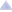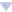### awoo's blog

By awoo, history, 5 years ago,1065A - Vasya and Chocolate

Tutorial
Solution (Ajosteen)

1065B - Vasya and Isolated Vertices

Tutorial
Solution (Ajosteen)

1065C - Make It Equal

Tutorial
Solution (Ajosteen)

1065D - Three Pieces

Tutorial
Solution (PikMike)

1065E - Side Transmutations

Tutorial
Solution (PikMike)

1065F - Up and Down the Tree

Tutorial

1065G - Fibonacci Suffix

Tutorial
Solution (BledDest)Comments (25)
| Write comment?
 » 44220948Why does my D WA on test 25? I had a similar solution, except I bruteforced the shortestdistance instead of using bds/djikstras, and used a bunch of selections for the dp instead of a pair.
•  » » 5 years ago, # ^ | ← Rev. 2 →   You probably didnt consider that in the optimal solution you need to change the chess piece on the way between position a and b and not only change pieces on position a or b?
•  » » » but a rook can always reach your desired position in 2 moves, so even if you switch to a rook at the beginning your total cost will be 3Now if you're using a bishop and move to a position which isn't your destination, and switch to another counter and then move to your desired position, your total cost would still be 3The same goes for switching between other counters. Your maximum cost will always be 3 (which is the same as switching to a rook at the beginning and then reaching your desired position)
•  » » » » 4 14 10 3 7 4 15 11 13 12 1 16 9 2 5 6 8 Consider, when you are in number 2 with a bishop. You can go to number 3 with a rook in 3 steps: replace bishop with rook, goto 14, goto number 3. You can go to number 3 with a knight in 3 steps: go back to 1 with bishop, replace bishop with knight, goto number 3. (Note that, you cannot replace bishop with knight in number 2 because, in that case, you need 4 steps to go to number 3)
•  » » » » Well, what if you number the positions as the positions of a knights tour? Then the optimal solution would be to start with a knight and the distance between each location would be 1.
 » 5 years ago, # | ← Rev. 2 →   O((N + M) * M) or O(M * (M + log(N))) solution for G:Same solution but changing 1 part, the part of counting the number of occurences. Instead of using an automaton, keep pref[i] ans suf[i] as the M first characters of the prefix/suffix of the i-th string. Using this, freq[i] = freq[i-2] + freq[i-1] + occurences that use the suf[i-2] + pref[i-1]. This gives us an O(N * M^2) solution like in the editorial. But you can note that starting from one point (the position where |F[i]| >= M), pref[i] == pref[i + 2] and suf[i] == suf[i + 1]. This means that the "occurences that use the suf[i-2] + pref[i-1]" will actually repeat with period 2. So you can calculate it in one phase until that point, the cost of this is O(F + F + F + ... + F[i]) = O(F[i + 1]) = O(M) and from that point on, you can reuse the number of occurences that happened in the previous calculations (or do 4 more just to be safe and use from that point on, doesn't change the complexity). Total complexity: O((N * M) * M) with one phase (additional character) happening in O(M + N) because you need to calculate the borders for KMP. You can actually use this fact to create a linear recurrence relation for freq[i] and use matrix exponentiation (the column matrix keeps freq[i], freq[i-1], the transitions and keep swapping them) starting from the point that it repeats, this would result in a O(log(M) + M + log(N) * 4^3) phase per character in the answer. The log(M) comes from the fact that fib is exponential, so the point that it starts repeating is O(log(M)). This would result in a O(M * (M + log(N))) solution.Code for O((N + M) * M) solution: http://codeforces.com/contest/1065/submission/44225229Edit: If there's an occurence in the transition, you can also keep calculating until it breaks K. It will break K in O(logK) steps and if there's no occurence, you just break. So I guess the second part wouldn't be necessary for the O(M^2) complexity.
•  » » 5 years ago, # ^ | ← Rev. 2 →   That also implies that a big N doesn't matter as can be seen here: http://codeforces.com/contest/1065/submission/44226008. n = min(n, 100) works. To be more exact, the bound on N mattering would be O(log(M) + log(K)).
 » https://codeforces.com/contest/1065/submission/44147964For C,my approach is a bit similar can anyone help me,where i am wrong?
 » Can someone explain d.
 » Hi! I have a problem can anybody help? if u submit this solution for problem D with MS C++ u get accept! Link to code! but gnu++ gives WA! do u know why?
 » Can E solve by polya?
•  » » Yes, you can. 44419650As the editorial said every subset of segments [0..l1), [l1, l2), …, [lm−1, lm) is achievable. There is a bijective relation between the set of subsets of segments and the set of transformations G and every transformation add An - k where k is the sum of the cardinalities of the segments in the associated subset.
•  » » » Yes! Actually, the formula solve by polya is the same as the official tuitual. Wonderful!
•  » » » » what is polya?
 » 5 years ago, # | ← Rev. 2 →   Why this this solution for problem 1065C is not correct ?Since the attainable equal height is the Smallest number.then Number of Chops can be : ceil((SumOfHeight - N * minHeight) / K)
•  » » aryan29's explanation is correct. Here, I'm providing a visual representation of a counter case so that people can get an intuitive understanding of the situation. Consider the test case: 3 3 5 3 1 Here ceil((SumOfHeight - N * minHeight) / K) will give 2 slices which is incorrect as we can not remove blocks from a height partially.Whereas the correct number of slices is 3.•  » » » 7 days ago, # ^ |0.ComplaintFrame { display: inline-block; position: absolute; top: 0; right: -1.4em; } .ComplaintFrame a { text-decoration: none; color: #ff8c00; opacity: 0.5; } .ComplaintFrame a:hover { opacity: 1; } ._ComplaintFrame_popup p, ._ComplaintFrame_popup button { margin-top: 1rem; } ._ComplaintFrame_popup input[type=submit] { padding: 0.25rem 2rem; } ._ComplaintFrame_popup ul { margin-top: 1em !important; margin-bottom: 1em !important; } thanks for solution, I learned something new...